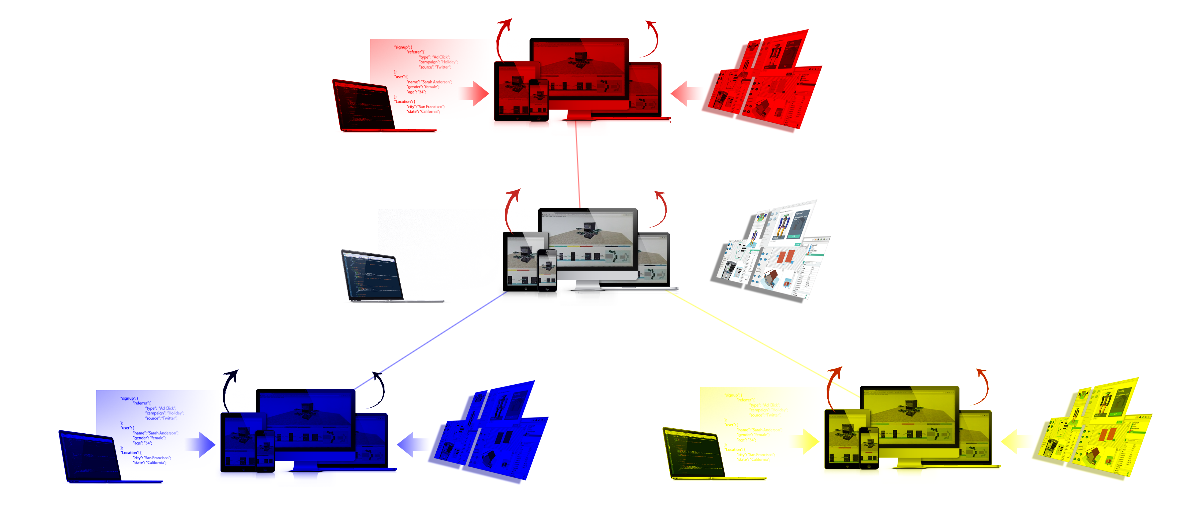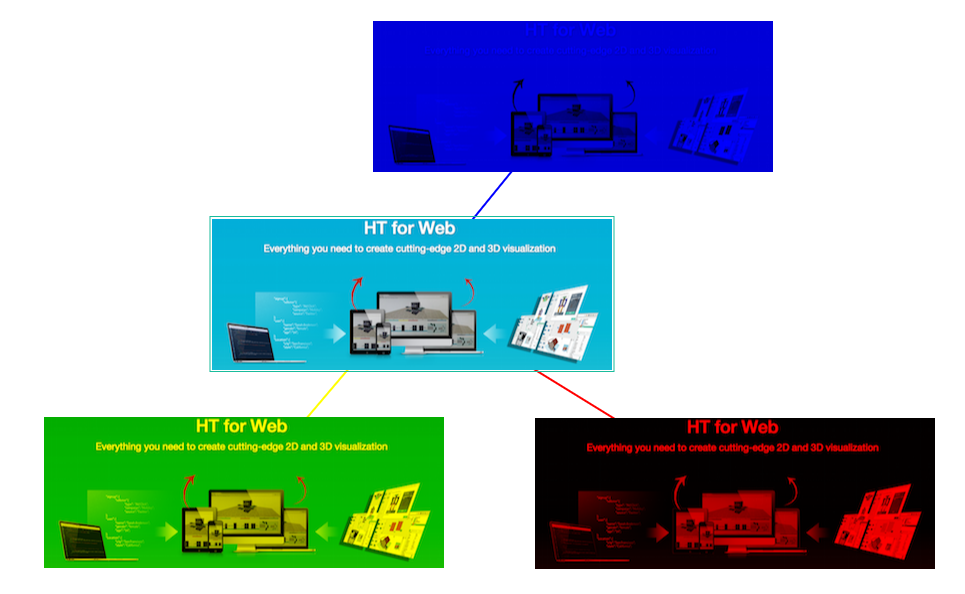Home » html5 » HT for Web基于HTML5的图像操作（一）

# HT for Web基于HTML5的图像操作（一）

HT for Web独创的矢量图片设计架构，使其具有强大丰富的动态图形呈现能力，但从最近知乎热议的“Adobe Photoshop 是否已经过时？”的话题，大家能体会到很多情况下实际项目不可能完全采用矢量，项目中还是有大量的传统非矢量的静态图片，毕竟工具强大且现有资源丰富，但为了使静态图片呈现出更多的动态效果，HT for Web提供了较多渲染方式，例如对HT for Web的图元设置‘body.color’的style后图元即可达到自动染色的效果，这里将对这种染色技术进行讲解。```dataModel = new ht.DataModel();
graphView = new ht.graph.GraphView(dataModel);
var root = new ht.Node();
root.setImage('ht-for-web.png');
['blue', 'yellow', 'red'].forEach(function(color){
var node = new ht.Node();
node.setImage('ht-for-web.png');
node.s({ 'body.color': color });
var edge = new ht.Edge(root, node);
edge.s({'edge.color': color});
});```

```	toRGBA = (function(){
var map = {}, // string - [128,128,128,255]
canvas;
return function(color){
var g, data = map[color];
if (!data) {
if(!canvas){
canvas = document.createElement('canvas');
canvas.width = 1;
canvas.height = 1;
}
g = canvas.getContext('2d');
g.clearRect(0, 0, 1, 1);
g.fillStyle = color;
g.beginPath();
g.rect(0, 0, 1, 1);
g.fill();
data = g.getImageData(0, 0, 1, 1).data;
data = map[color] = [data, data, data, data];
}
return data;
};
})();

function init(){
var image = new Image();
image.src = 'banner.png';// 'ht-for-web.png';
dataModel = new ht.DataModel();
graphView = new ht.graph.GraphView(dataModel);

var root = new ht.Node();
root.setImage(image);

['blue', 'yellow', 'red'].forEach(function(color){
var node = new ht.Node();
node.setImage(createColorImage1(image, color));
var edge = new ht.Edge(root, node);
edge.s({
'edge.color': color
});
});
};
}
function createColorImage1(image, color) {
var width = image.width;
var height = image.height;
var canvas = document.createElement('canvas');
var context = canvas.getContext( "2d" );
canvas.width = width;
canvas.height = height;
context.drawImage(image, 0, 0, width, height);
var rgba = toRGBA(color);
var r = rgba/255, g = rgba/255, b = rgba/255;
var pixelData = context.getImageData(0, 0, width, height);
var pixels = pixelData.data;
for (var i = 0; i < pixels.length; i += 4)
{
pixels[i+0] *= r;
pixels[i+1] *= g;
pixels[i+2] *= b;
}
context.putImageData(pixelData, 0, 0);
return canvas;
}```